Next: Worked example 6.4: Bullet Up: Conservation of momentum Previous: Worked example 6.2: Hitting

## Worked example 6.3: Skater and medicine ball

Question: A skater of mass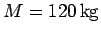is skating across a pond with uniform velocity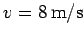. One of the skater's friends, who is standing at the edge of the pond, throws a medicine ball of mass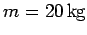with velocity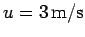to the skater, who catches it. The direction of motion of the ball is perpendicular to the initial direction of motion of the skater. What is the final speed of the skater? What is the final direction of motion of the skater relative to his/her initial direction of motion? Assume that the skater moves without friction.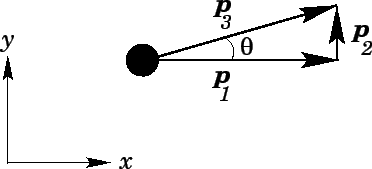Answer: Suppose that the skater is initially moving along the-axis, whereas the initial direction of motion of the medicine ball is along the-axis. The skater's initial momentum is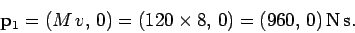Likewise, the initial momentum of the medicine ball is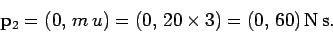After the skater catches the ball, the combined momentum of the skater and the ball is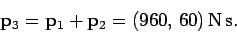This follows from momentum conservation. The final speed of the skater (and the ball) is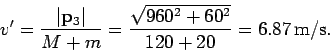The final direction of motion of the skater is parameterized by the angle(see the above diagram), where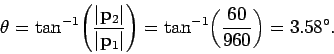Next: Worked example 6.4: Bullet Up: Conservation of momentum Previous: Worked example 6.2: Hitting
Richard Fitzpatrick 2006-02-02